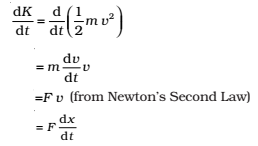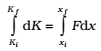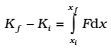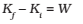The work-energy theorem states that the change in kinetic energy of a body is the work done by the net force on the body.

Kf – Ki = Wnet

## Work-energy theorem for a Variable Force:

We are now familiar with the concepts of work and kinetic energy to prove the work-energy theorem for a variable force. We confine ourselves to one dimension. The time rate of change of kinetic energy isThus,

dK = Fdx

Integrating from the initial position (xi) to final position (xf), we havewhere, Ki and Kf are the initial and final kinetic energies corresponding to xi and xf .

ororThus, the WE theorem is proved for a variable force.

The WE theorem is not independent of Newton’s Second Law. The WE theorem may be viewed as a scalar form of the Second Law. The principle of conservation of mechanical energy may be viewed as a consequence of the WE theorem for conservative forces.

The WE theorem holds in all inertial frames. It can also be extended to non inertial frames provided we include the pseudo forces in the calculation of the net force acting on the body under consideration.